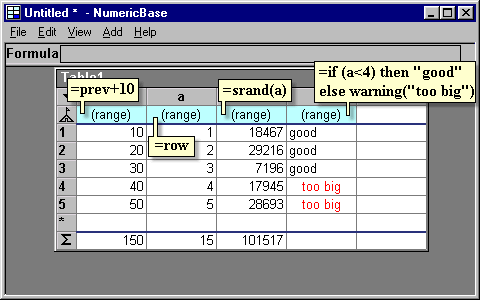# Functions

Functions
NumericBase let you combine function calls in you formulas. You can either use the build-in functions, or create new ones yourself.

Some build-in functions let you perform various summary calculations on you data, such as summary, average, sorting, etc.

The function call syntax
A function call has two elements that must be in the following order:
1. Function name. Must be a "simple name" - any combination of digits, letters, the underscore sign (_), and  the tilde sign (~), that does not begin with a digit.
2. Argument list - a sequence of zero or more formulas separated by commas, and surrounded by parentheses.
Example for a function call: =function_name(4,45)

Function with zero augments
If the function has zero augments, then you can omit the argument list: for example func_name is equivalent to func_name().

Build in functions.
NumericBase comes with a some build-in functions. These functions perform the standard mathematical functions such as sinus, cosine, logarithm , etc. Other build-in functions perform operations that are unique to NumericBase.

The following table shows the functions that are unique to NumericBase.

 Build&-in function. Meaning row() Returns the row number. col() Returns the column number. default() Returns the default value of the cell. (planned) prev() Return the value of the cell at the prev row and the same column. next() Returns the value of the cell at the next row and the same column. srand(seed) Returns a pseudo number based on the seed. You can use this to generate pseudo number sequences that do not change from one calculation to another. warning(message) Creates a warning.
Table: functions unique to NumericBase.

Some of the build-in functions are demonstrated in the following screenshot:Screenshot: some of the build-in functions

Implicit range
Some functions, such as row and prev, can not be calculated at the base row. When you use these functions at the base row, as in the above screenshot, the functions evaluate to a (range) message. This is called an implicit range because, like in range formulas, it produces different values across the rows of the table.